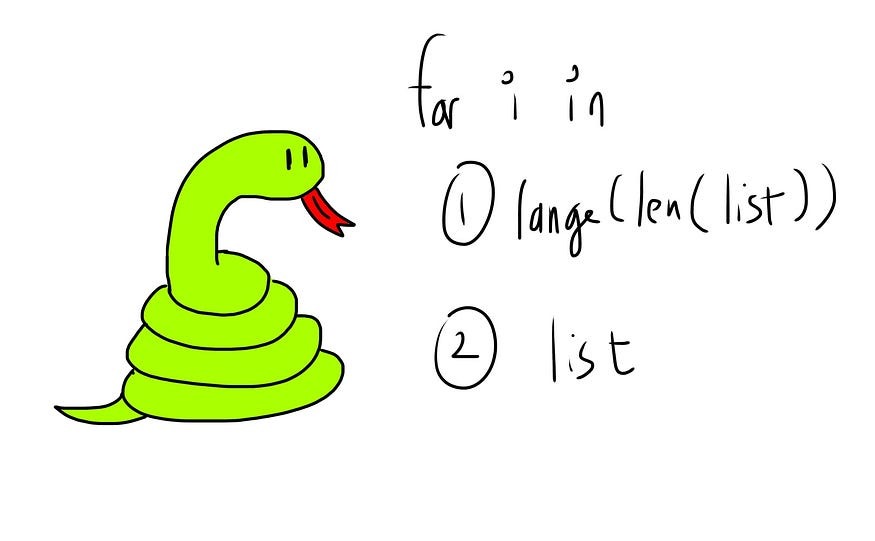# “for i in range(len(list))” VS “for element in list” — When to Use Which

## A beginner's guide on the two ways of iterating through a string or list.If you’re just starting out in Python, the for loop is one of the most important concepts you need to get familiar with. When iterating through a string or list, there are 2 ways to do this:

``````list1 = ["apple", "orange", "pear"]# method 1
for i in range(len(list1))# method 2
for element in list1
``````

### Method 1 — for i in range(len(list1))

Here, `len(list1)` will return 3, and input 3 into the `range` function generates the indexes of the stuff inside the list (known as elements).

``````for i in range(len(list1)):
print(i)# same as for i in range(3)
``````

Here, `range(len(list1))` is the same as `range(3)`, so the iterator variable `i` is assigned the values 0, 1 then 2. The output:

``````0
1
2
``````

To get our element, we simply need to index our list using `i`.

``````for i in range(len(list1)):
print(i, list1[i])
``````

The output:

``````0 apple
1 orange
2 pear
``````

### Method 2 — for element in list1

Here, we loop through the list directly instead of the list’s index.

``````for element in list1:
print(element)
``````

The iterator variable `element` (note that you can use whatever variable name you want) is thus assigned the elements in the list, and takes on the values `"apple"`, `"orange"` then `"pear"`. The output:

``````apple
orange
pear
``````

Note that if we use this method, we are unable to get the index of the elements. (Don’t use the .index(element) method, as it finds only the first-found element)

### The Main Difference

``````# method 1
for i in range(len(list1)):
print(i, list1[i])# method 2
for element in list1:
print(element)
``````

Here, the main difference is that we can get the index of each element in method 1, but not in method 2. (We need to type less code using method 2, so that’s a plus)

### When To Use Method 2 — for element in a list

We use this when we have no need for the index of the elements inside the list. For instance:

Finding the sum of odd numbers in a list:

``````lis = [1,2,3,4,5,6]total = 0
for number in lis:
if number%2==1:
total += number
``````

Finding squares of numbers in a list:

``````lis = [1,2,3,4,5,6]output = []
for number in lis:
output.append(number**2)
``````

These can be solved also using method 1 (`for i in range(len(list))`), but we don’t necessarily have to. We can simply choose to write a little less code and use method 2 (`for element in list`), and we’ll still be able to solve these.

### When To Use Method 1 — for i in range(len(list))

We use this method when we need the index of the elements — especially when we need more than 1 index per iteration. For instance:

Finding the sum of consecutive numbers:

``````lis = [1,10,100,1000,10000]output = []
for i in range(len(lis)-1):
this = lis[i]
next = lis[i+1]
output.append(this+next)# [11,110,1100,11000]
``````

Combining consecutive strings together:

``````lis = ["apple", "orange", "pear", "durian"]output = []
for i in range(len(lis)-1):
this = lis[i]
next = lis[i+1]
output.append(this + " " + next)# ["apple orange", "orange pear", "pear durian"]
``````

Finding numbers smaller than both left & right neighbours:

``````lis = [5,3,4,6,2,7,1,8]output = []
for i in range(len(lis)-2):
left = lis[i]
this = lis[i+1]
right = lis[i+2]
if this < left and this < right:
output.append(this)# [3,2,1]
``````

These problems can only be solved using method 1 (`for i in range(len(list))`) but not method 2 (`for element in list`), as we must have the index of each element to get more than 1 element at each iteration.